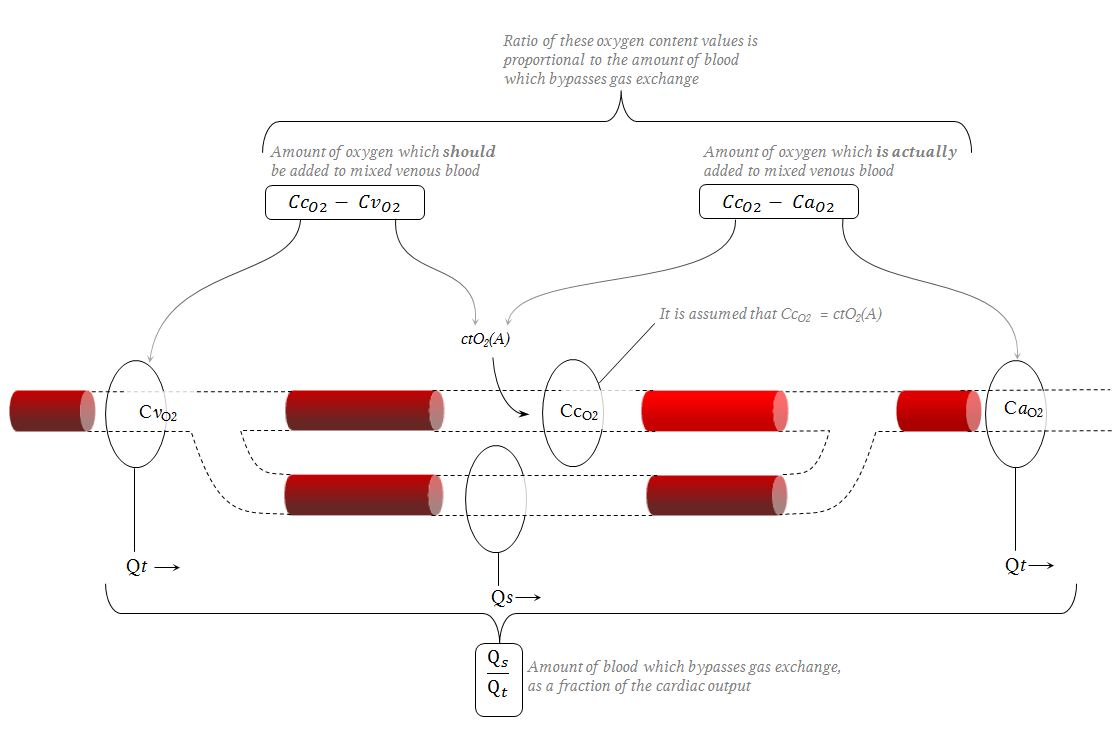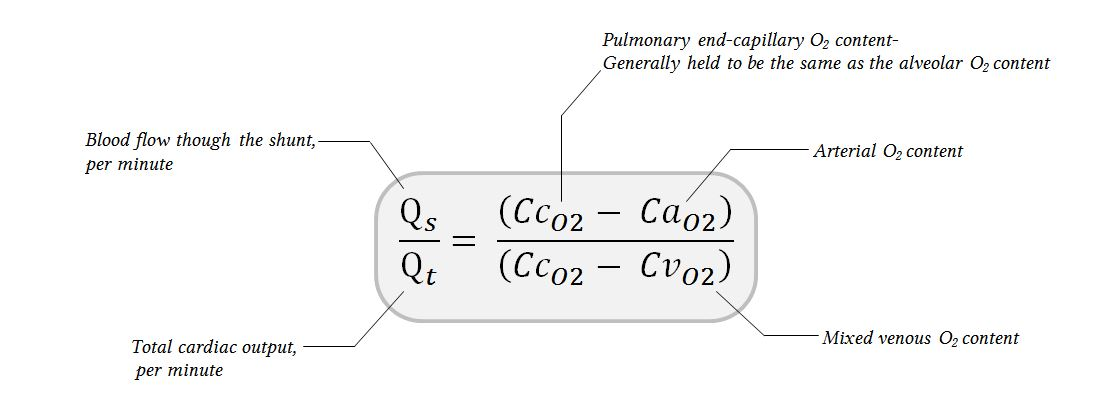# Measurement and estimation of shunt

This chapter is most relevant to Section F6(vi) from the 2017 CICM Primary Syllabus, which expects the exam candidates to be able to "explain the concept of shunt, its physiological effects and its measurement". The concept of shunt and venous admixture being explained elsewhere, this chapter will focus on the "its measurement" part of the syllabus. This discussion is made more difficult as in actual fact shunt cannot be measured directly, and so all such "measurements" are actually calculating venous admixture.

In summary:

• The shunt equation, otherwise known as the Berggren equation, is used to calculate the shunt fraction:
Qs/Qt = (CcO2 - CaO2) / (CcO2 - CvO2)
where
Qs/Qt = shunt fraction (shunt flow divided by total cardiac output)
CcO2 = pulmonary end-capillary O2 content, same as alveolar O2 content
CaO2 = arterial O2 content
CvO2 = mixed venous O2 content
• The estimated shunt fraction (Fshunte) can be calculated if an assumed value is substituted for CvO2) in the Berggren equation
• "True" shunt can be identified if the subject is made to breathe 100% FiO2
• This decreases the contribution from V/Q scatter
• With 100% FiO2, the measured shunt fraction is the "true" intrapulmonary shunt
• This technique does not separate "true" shunt from anatomical shunt (contribution from thebesian veins and bronchial veins) or cardiac defects

The best free reference is Bigeleisen (2001), which is an excellent resource to "help students master these equations as well as their practical limitations".

## The shunt equation

Confusingly, the shunt equation is used to estimate the venous admixture, relying on a model of the lung which divides it conceptually into regions with V/Q of 1 and regions with V/Q of 0.

As always, these things are easier to represent as a big confusing diagram.Let us dissect these variables.

• CvO2, the oxygen content of mixed venous blood, is a known variable (as you can measure it), and it is returning to the lungs at a flow rate equal to the cardiac output, Qt.
• CcO2 is the oxygen content of pulmonary endcapillary blood, and it is assumed that it is equal to CtO2(A), the alveolar oxygen content. This assumption is based on a two-compartment model, where this compartment is perfectly oxygenated (i.e. the arterial and alveolar oxygen content is the same).
•  (CcO2 - CvO2) is the difference in oxygen content between mixed venous and "perfect" endcapillary blood
• Qs is the flow through the shunt fraction, which is returning perfectly unchanged mixed venous blood back to the systemic arterial circulation
• CaO2 is the oxygen content of systemic arterial blood, which will be lower than the content of "perfect" endcapillary blood because it is mixed with the relatively hypoxic Qs.
• (CcO2 - CaO2) is the difference in oxygen content between "perfect" endcapillary blood and systemic arterial blood; this drop in oxygen content is due to the venous admixture.

Of these quantities, all are known except the Qs, which is the shunt fraction. Well, the pulmonary end-capillary oxygen content is not known, but it is assumed. Obviously nobody is ever going to get into those capillaries and measure it directly. Instead an assumption is made that the ventilated areas of the lung are perfused with "ideal" capillaries, and the gas exchange in them is so perfect that their end-capillary oxygen tension is equal to alveolar oxygen tension, and their saturation is 100%.

Determining Qs is therefore a matter of subtracting (CcO2 + CvO2) from CaO2. However, we don't know what the flow is. We can only say that if all blood were ideally oxygenated the CaO2 should be the same as CcO2, and if all blood were completely shunted the CaO2 should be the same as CvO2.

The relationship between the different fractions must therefore remain a ratio, rather than a real oxygen difference in ml. Hence the shunt fraction is usually represented as Qs/Qt.

The famous relationship below which determines this ratio is the so-called Berggren equation, which is usually referred to as the "shunt equation" (Sven M. Berggren, 1942).  The equation compares arterial oxygen content to pulmonary end-capillary oxygen content (CcO2):Thus, we can plug in some values, for a typical hypoxic ICU patient with an arterial saturation of 90%, and a decently anaemic Hb of 100g/L. The oxygen content of blood is calculated as (sO2 × ceHb × 1.39) + (PaO2 × 0.03); though usually people will use 1.3 or 1.34 as these are more "realistic" BOvalues, and ctHb if the ceHb is not available (because the normal fractions of dyshaemoglobins are in the 1-2% range, and can be safely ignored). The contribution of dissolved oxygen can also be safely ignored, as it is trivial. This solubility coefficient is variably expressed as 0.03ml/L/mmHg (if you use a haemoglobin concentration measured in g/L) or 0.003ml/100ml/mmHg (if you're using haemoglobin measured in g/dL as in this equation from Cornell).

Conceptually, we can represent the output of these calculations as a volume of systemic arterial blood which is separated into two compartments, one of which is fully oxygenated and one which is fully composed of unchanged venous blood:Thus, the mixed venous blood content is 97.5ml/L, and the fully oxygenated end-capilary blood content is 130ml/L. The shunt equation can now be used to determine how much mixed venous blood one would need to add to the capillary blood to create the systemic arterial oxygen content, which is 117ml/L:

Qs/Qt = (130-117) / ( 130 - 97.5) = 0.4

Thus, in this example, the shunt fraction is 40%.

## The estimated shunt fraction, FShunte

The local ABG machine may be capable of producing some estimate of shunt without any direct measurement of mixed venous oxygen content. It is possible to make certain assumptions about the mixed venous blood, and substitute those assumptions into the shunt equation.  The ABG machine can do this calculation on our behalf; the result is usually reported as FShunte where the little "e" indicates "estimate".

Recall the Berggren equation:The local ABG machine rearranges the Berggren equation, and uses a slightly different nomenclature.All of the variables used in this equation are measured in the same ABG, with the exception of the O2 content of alveolar air, and you can get that from the alveolar gas equation.

## Assumptions used in calculating the FShunte

In the absence of mixed venous data, the blood gas analyser uses an assumed veno-arterial oxygen content difference. The assumed difference is 2.3mmol/L, according to the reference manual for the local ABG machine.Let us look at that assumption.

2.3 mmol/L of oxygen seems like a very small difference, but actually it is not.

An easily available online ideal gas law calculator reveals that under standard conditions 2.3mmol of oxygen equates to about 51ml/L, using whatever equation Radiometer happened to use. Now, if the arterial blood is assumed to contain 150g/L of maximally oxygenated haemoglobin, then its oxygen content is actually about 208.8ml/L. The content of venous blood therefore must be about 157.8ml/L. Running this backwards through the DO2 equation, one discovers that an oxygen saturation of around 77% is required, which just so happens to be the high end of average mixed venous oxygen saturation.

From this stems the greatest critique of the estimated FShunte. The ICU patients can vary greatly in their mixed venous oxygen saturation, from the cyanide-poisoned patient with an SvO2 of 90%, to the severe sepsis patient with an SvO2 of 30%. In these two examples, the venoarterial oxygen differences would be 10ml/L and 145.9ml/L, respectively. Obviously, making assumptions about the mixed venous saturation of critically ill patients will lead to errors, as most of them will not have an SvO2 of 77%

Additionally, a criticism of all shunt calculations is the fact that they use a two-compartment model. If the FShunt is 25%, the equation leads one to believe that in a two-compartment lung, 25% of the blood is travelling through the non-ventilated compartment. In reality, the lung is a mixture of heterogeneous units, each with a different V/Q ratio. The shunt equation is therefore a very gross estimate of oxygenation defects.

## Elimination of V/Q scatter: measuring shunt with 100% FiO2

Let us say one has encountered a sizeable difference between the arterial and alveolar oxygen content. If one were for some reason in need of estimating how much of that difference comes from "true" shunt and how much comes from V/Q scatter, one could use the MIGET technique, which is the gold standard but quite technically complex, or one could see what happens when one increases the FiO2. V/Q scatter and "true" shunt react quite differently to increases in allveolar oxygen concentration.

As the FiO2 increases, areas of low V/Q ratio would suddenly find themselves ventilated with a higher oxygen concentration. Even small increases in oxygen concentration here will yield substantial increases if endcapillary oxygenation, because of the steepness of the oxygen-haemoglobin dissociation curve. Thus, the difference between endcapillary blood and arterial blood would diminish, such that at 100% FiO2 the contribution of V/Q scatter would be minimal. Sure, there would still be lung units with an extremely low V/Q ratio (less than 0.10), but these are usually few, and the amount of blood passing through them would be minimal (ergo their contribution to arterial blood would also be small). However, for lung units with "true" shunt, V/Q = 0, and no amount of extra inspired oxygen is going to improve their oxygenation. As a consequence of this, breathing a high FiO2 essentially eliminates the contribution of V/Q scatter to the total shunt fraction, and what is left over is the "true" shunt.

## References

Said, Sami I., and Chandra M. Banerjee. "Venous admixture to the pulmonary circulation in human subjects breathing 100 per cent oxygen." The Journal of clinical investigation 42.4 (1963): 507-515.

Sven M. Berggren. "The Oxygen Deficit of Arterial Blood Caused by Non-ventilating Parts of the Lung" 1942; Volume 11 of Acta Physiologica Scandinavica: 4; Supplementum 11.

Bigeleisen, Paul E. "Models of venous admixture.Advances in physiology education 25.3 (2001): 159-166.